## Section Exercises

1. Explain the difference between the coefficient of a power function and its degree.

2. If a polynomial function is in factored form, what would be a good first step in order to determine the degree of the function?

3. In general, explain the end behavior of a power function with odd degree if the leading coefficient is positive.

4. What is the relationship between the degree of a polynomial function and the maximum number of turning points in its graph?

5. What can we conclude if, in general, the graph of a polynomial function exhibits the following end behavior? As $x\to -\infty ,f\left(x\right)\to -\infty$ and as $x\to \infty ,f\left(x\right)\to -\infty$.

For the following exercises, identify the function as a power function, a polynomial function, or neither.

6. $f\left(x\right)={x}^{5}$

7. $f\left(x\right)={\left({x}^{2}\right)}^{3}$

8. $f\left(x\right)=x-{x}^{4}$

9. $f\left(x\right)=\frac{{x}^{2}}{{x}^{2}-1}$

10. $f\left(x\right)=2x\left(x+2\right){\left(x - 1\right)}^{2}$

11. $f\left(x\right)={3}^{x+1}$

For the following exercises, find the degree and leading coefficient for the given polynomial.

12. $-3x{}^{4}$

13. $7 - 2{x}^{2}$

14. $-2{x}^{2}- 3{x}^{5}+ x - 6$

15. $x\left(4-{x}^{2}\right)\left(2x+1\right)$

16. ${x}^{2}{\left(2x - 3\right)}^{2}$

For the following exercises, determine the end behavior of the functions.

17. $f\left(x\right)={x}^{4}$

18. $f\left(x\right)={x}^{3}$

19. $f\left(x\right)=-{x}^{4}$

20. $f\left(x\right)=-{x}^{9}$

21. $f\left(x\right)=-2{x}^{4}- 3{x}^{2}+ x - 1$

22. $f\left(x\right)=3{x}^{2}+ x - 2$

23. $f\left(x\right)={x}^{2}\left(2{x}^{3}-x+1\right)$

24. $f\left(x\right)={\left(2-x\right)}^{7}$

For the following exercises, find the intercepts of the functions.

25. $f\left(t\right)=2\left(t - 1\right)\left(t+2\right)\left(t - 3\right)$

26. $g\left(n\right)=-2\left(3n - 1\right)\left(2n+1\right)$

27. $f\left(x\right)={x}^{4}-16$

28. $f\left(x\right)={x}^{3}+27$

29. $f\left(x\right)=x\left({x}^{2}-2x - 8\right)$

30. $f\left(x\right)=\left(x+3\right)\left(4{x}^{2}-1\right)$

For the following exercises, determine the least possible degree of the polynomial function shown.

31.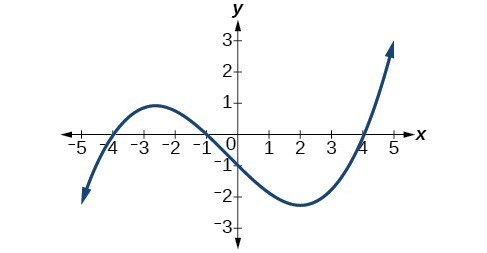32.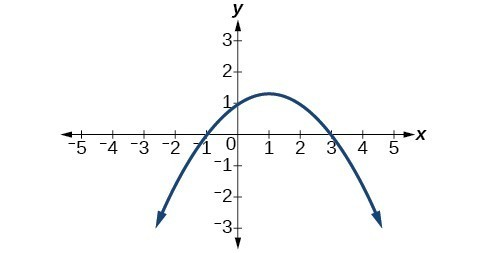33.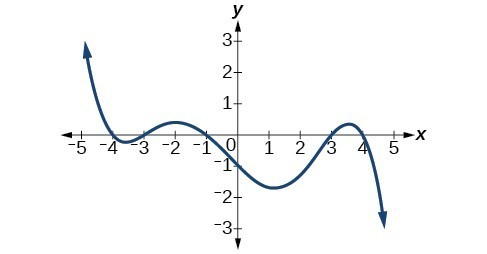34.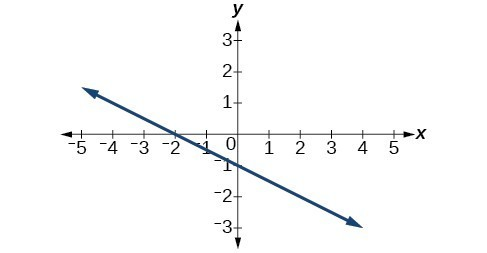35.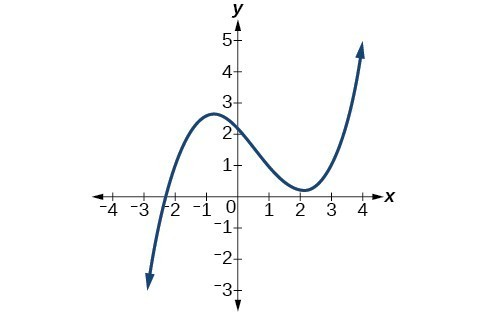36.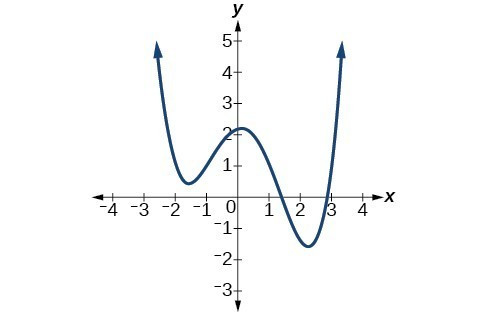37.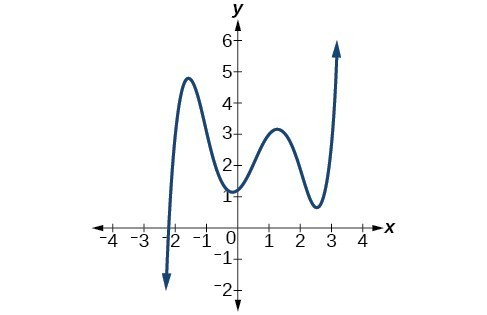38.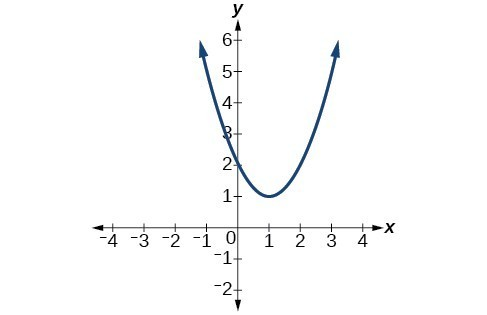For the following exercises, determine whether the graph of the function provided is a graph of a polynomial function. If so, determine the number of turning points and the least possible degree for the function.

39.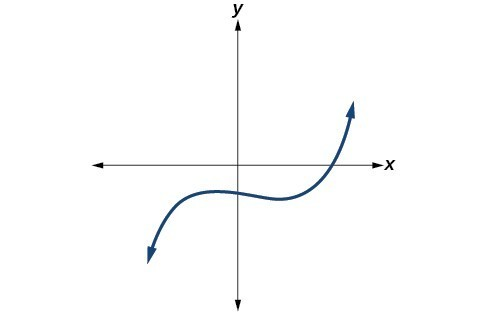40.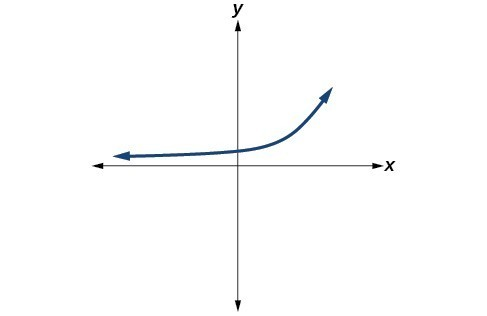41.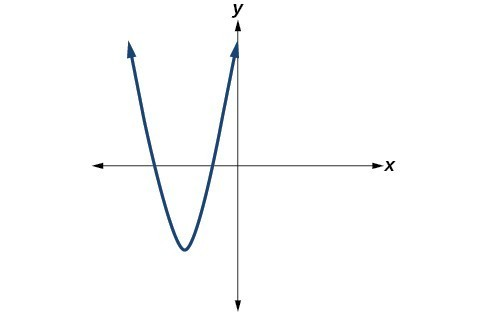42.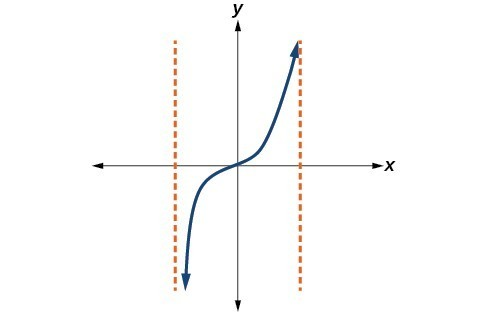43.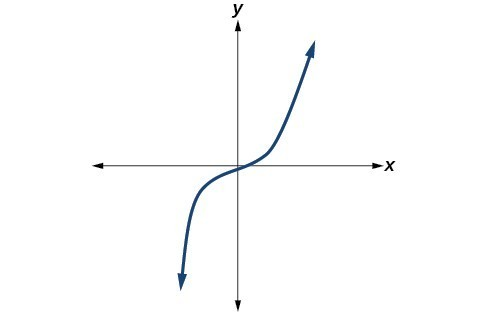44.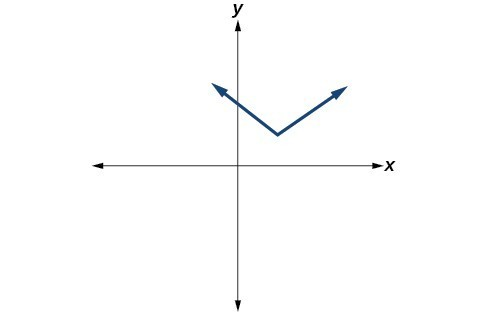45.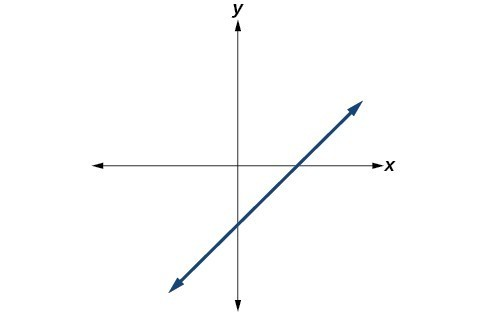For the following exercises, make a table to confirm the end behavior of the function.

46. $f\left(x\right)=-{x}^{3}$

47. $f\left(x\right)={x}^{4}-5{x}^{2}$

48. $f\left(x\right)={x}^{2}{\left(1-x\right)}^{2}$

49. $f\left(x\right)=\left(x - 1\right)\left(x - 2\right)\left(3-x\right)$

50. $f\left(x\right)=\frac{{x}^{5}}{10}-{x}^{4}$

For the following exercises, graph the polynomial functions using a calculator. Based on the graph, determine the intercepts and the end behavior.

51. $f\left(x\right)={x}^{3}\left(x - 2\right)$

52. $f\left(x\right)=x\left(x - 3\right)\left(x+3\right)$

53. $f\left(x\right)=x\left(14 - 2x\right)\left(10 - 2x\right)$

54. $f\left(x\right)=x\left(14 - 2x\right){\left(10 - 2x\right)}^{2}$

55. $f\left(x\right)={x}^{3}-16x$

56. $f\left(x\right)={x}^{3}-27$

57. $f\left(x\right)={x}^{4}-81$

58. $f\left(x\right)=-{x}^{3}+{x}^{2}+2x$

59. $f\left(x\right)={x}^{3}-2{x}^{2}-15x$

60. $f\left(x\right)={x}^{3}-0.01x$

For the following exercises, use the information about the graph of a polynomial function to determine the function. Assume the leading coefficient is 1 or –1. There may be more than one correct answer.

61. The y-intercept is $\left(0,-4\right)$. The x-intercepts are $\left(-2,0\right),\left(2,0\right)$. Degree is 2.

End behavior: $\text{as }x\to -\infty ,f\left(x\right)\to \infty ,\text{ as }x\to \infty ,f\left(x\right)\to \infty$.

62. The y-intercept is $\left(0,9\right)$. The x-intercepts are $\left(-3,0\right),\left(3,0\right)$. Degree is 2.

End behavior: $\text{as }x\to -\infty ,f\left(x\right)\to -\infty ,\text{ as }x\to \infty ,f\left(x\right)\to -\infty\\$.

63. The y-intercept is $\left(0,0\right)$. The x-intercepts are $\left(0,0\right),\left(2,0\right)$. Degree is 3.

End behavior: $\text{as }x\to -\infty ,f\left(x\right)\to -\infty ,\text{ as }x\to \infty ,f\left(x\right)\to \infty$.

64. The y-intercept is $\left(0,1\right)$. The x-intercept is $\left(1,0\right)$. Degree is 3.

End behavior: $\text{as }x\to -\infty ,f\left(x\right)\to \infty ,\text{ as }x\to \infty ,f\left(x\right)\to -\infty$.

65. The y-intercept is $\left(0,1\right)$. There is no x-intercept. Degree is 4.

End behavior: $\text{as }x\to -\infty ,f\left(x\right)\to \infty ,\text{ as }x\to \infty ,f\left(x\right)\to \infty$.

For the following exercises, use the written statements to construct a polynomial function that represents the required information.

66. An oil slick is expanding as a circle. The radius of the circle is increasing at the rate of 20 meters per day. Express the area of the circle as a function of d, the number of days elapsed.

67. A cube has an edge of 3 feet. The edge is increasing at the rate of 2 feet per minute. Express the volume of the cube as a function of m, the number of minutes elapsed.

68. A rectangle has a length of 10 inches and a width of 6 inches. If the length is increased by x inches and the width increased by twice that amount, express the area of the rectangle as a function of x.

69. An open box is to be constructed by cutting out square corners of x-inch sides from a piece of cardboard 8 inches by 8 inches and then folding up the sides. Express the volume of the box as a function of x.

70. A rectangle is twice as long as it is wide. Squares of side 2 feet are cut out from each corner. Then the sides are folded up to make an open box. Express the volume of the box as a function of the width (x).